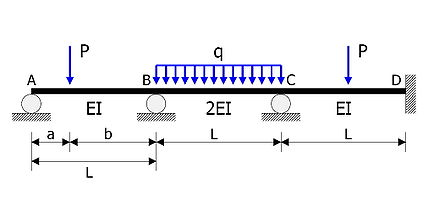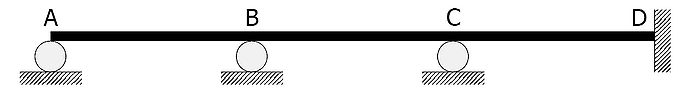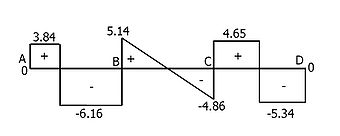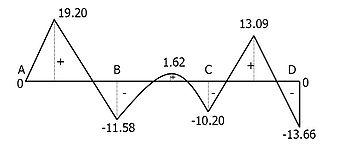# Moment distribution method

Moment distribution method

The moment distribution method (not to be confused with moment redistribution) is a structural analysis method for statically indeterminate beams and frames developed by Hardy Cross. It was published in 1930 in an ASCE journal. The method only accounts for flexural effects and ignores axial and shear effects. From the 1930s until computers began to be widely used in the design and analysis of structures, the moment distribution method was the most widely practiced method.

## Introduction

In the moment distribution method, every joint of the structure to be analysed is fixed so as to develop the fixed-end moments. Then each fixed joint is sequentially released and the fixed-end moments (which by the time of release are not in equilibrium) are distributed to adjacent members until equilibrium is achieved. The moment distribution method in mathematical terms can be demonstrated as the process of solving a set of simultaneous equations by means of iteration.

The moment distribution method falls into the category of displacement method of structural analysis.

## ExampleExample

The statically indeterminate beam shown in the figure is to be analysed.

• Members AB, BC, CD have the same length$L = 10 \ m$.
• Flexural rigidities are EI, 2EI, EI respectively.
• Concentrated load of magnitude$P = 10 \ kN$ acts at a distance$a = 3 \ m$ from the support A.
• Uniform load of intensity$q = 1 \ kN/m$ acts on BC.
• Member CD is loaded at its midspan with a concentrated load of magnitude$P = 10 \ kN$.

In the following calcuations, counterclockwise moments are positive.

### Fixed end moments$M _{AB} ^f = \frac{Pb^2a }{L^2} = \frac{10 \times 7^2 \times 3}{10^2} = + 14.700 \ kN\cdot m$$M _{BA} ^f = - \frac{Pa^2b}{L^2} = - \frac{10 \times 3^2 \times 7}{10^2} = - 6.300 \ kN\cdot m$$M _{BC} ^f = \frac{qL^2}{12} = \frac{1 \times 10^2}{12} = + 8.333 \ kN\cdot m$$M _{CB} ^f = - \frac{qL^2}{12} = - \frac{1 \times 10^2}{12} = - 8.333 \ kN\cdot m$$M _{CD} ^f = \frac{PL}{8} = \frac{10 \times 10}{8} = + 12.500 \ kN\cdot m$$M _{DC} ^f = - \frac{PL}{8} = - \frac{10 \times 10}{8} = - 12.500 \ kN\cdot m$

### Flexural stiffness and distribution factors

The flexural stiffness of members AB, BC and CD are$\frac{3EI}{L}$,$\frac{4\times 2EI}{L}$ and$\frac{4EI}{L}$, respectively. Therefore:$D_{BA} = \frac{\frac{3EI}{L}}{\frac{3EI}{L}+\frac{4\times 2EI}{L}} = \frac{\frac{3}{10}}{\frac{3}{10}+\frac{8}{10}} = 0.2727$$D_{BC} = \frac{\frac{4\times 2EI}{L}}{\frac{3EI}{L}+\frac{4\times 2EI}{L}} = \frac{\frac{8}{10}}{\frac{3}{10}+\frac{8}{10}} = 0.7273$$D_{CB} = \frac{\frac{4\times 2EI}{L}}{\frac{4\times 2EI}{L}+\frac{4EI}{L}} = \frac{\frac{8}{10}}{\frac{8}{10}+\frac{4}{10}} = 0.6667$$D_{CD} = \frac{\frac{4EI}{L}}{\frac{4\times 2EI}{L}+\frac{4EI}{L}} = \frac{\frac{4}{10}}{\frac{8}{10}+\frac{4}{10}} = 0.3333$

The distribution factors of joints A and D are DAB = 1 and DDC = 0.

### Carryover factors

The carryover factors are$\frac{1}{2}$, except for the carryover factor from B to A which is zero.

### Moment distributionJoint A Joint B Joint C Joint D
Distrib. factors 0 1 0.2727 0.7273 0.6667 0.3333 0 0
Fixed-end moments 14.700 -6.300 8.333 -8.333 12.500 -12.500
Step 1 -14.700 -7.350
Step 2 1.450 3.867 1.934
Step 3 -2.034 -4.067 -2.034 -1.017
Step 4 0.555 1.479 0.739
Step 5 -0.246 -0.493 -0.246 -0.123
Step 6 0.067 0.179 0.090
Step 7 -0.030 -0.060 -0.030 -0.015
Step 8 0.008 0.022 0.011
Step 9 -0.004 -0.007 -0.004 -0.002
Step 10 0.001 0.003
Sum of moments 0 -11.569 11.569 -10.186 10.186 -13.657

Numbers in grey are balanced moments; arrows ( → / ← ) represent the carry-over of moment from one end to the other end of a member.

• Step 1
As joint A is released, balancing moment of magnitude equal to the fixed end moment$M_{AB}^{f} = 14.700 \mathrm{\,kN \,m}$ develops and is carried-over from joint A to joint B.
• Step 2
The unbalanced moment at joint B now is the summation of the fixed end moments$M_{BA}^{f}$,$M_{BC}^{f}$ and the carry-over moment from joint A. This unbalanced moment is distributed to members BA and BC in accordance with the distribution factors DBA = 0.2727 and DBC = 0.7273. Step 2 ends with carry-over of balanced moment$M_{BC}=3.867 \mathrm{\,kN \,m}$ to joint C. Joint A is a roller support which has no rotational restraint, so moment carryover from joint B to joint A is zero.
• Step 3
The unbalanced moment at joint C now is the summation of the fixed end moments$M_{CB}^{f}$,$M_{CD}^{f}$ and the carryover moment from joint B. As in the previous step, this unbalanced moment is distributed to each member and then carried over to joint C and back to joint B. Joint D is a fixed support and carried-over moments to this joint will not be distributed nor be carried over to joint C.
• Step 4
Joint B still has unbalanced moment which was carried over from joint C in step 3. Joint B is released once again to induce moment distribution and to achieve equilibrium.
• Steps 5 - 10
Joints are released and fixed again until every joint has unbalanced moments of size zero or neglectably small in required precision. Arithmetically summing all moments in each respective columns gives the final moment values.

### Result

• Moments at joints determined by the moment distribution method$M_A = 0 \ kN \cdot m$$M_B = -11.569 \ kN \cdot m$$M_C = -10.186 \ kN \cdot m$$M_D = -13.657 \ kN \cdot m$
The conventional engineer's sign convention is used here, i.e. positive moments cause elongation at the bottom part of a beam member.

For comparison purposes, the following are the results generated using a matrix method. Note that in the analysis above, the iterative process was carried to >0.01 precision. The fact that the matrix analysis results and the moment distribution analysis results match to 0.001 precision is mere coincidence.

• Moments at joints determined by the matrix method$M_A = 0 \ kN \cdot m$$M_B = -11.569 \ kN \cdot m$$M_C = -10.186 \ kN \cdot m$$M_D = -13.657 \ kN \cdot m$

The complete shear and bending moment diagrams are as shown. Note that the moment distribution method only determines the moments at the joints. Developing complete bending moment diagrams require additional calculations using the determined joint moments and internal section equilibrium.

• SFD and BMDShear force diagramBending moment diagram

Wikimedia Foundation. 2010.

### Look at other dictionaries:

• Method of moments — may refer to: Method of moments (statistics), a method of parameter estimation in statistics Method of moments (probability theory), a way of proving convergence in distribution in probability theory Second moment method, a technique used in… …   Wikipedia

• Moment (mathematics) — Second moment redirects here. For the technique in probability theory, see Second moment method. See also: Moment (physics) Increasing each of the first four moments in turn while keeping the others constant, for a discrete uniform distribution… …   Wikipedia

• Method of moments (statistics) — See method of moments (probability theory) for an account of a technique for proving convergence in distribution. In statistics, the method of moments is a method of estimation of population parameters such as mean, variance, median, etc. (which… …   Wikipedia

• Method of image charges — The method of image charges (also known as the method of images and method of mirror charges) is a basic problem solving tool in electrostatics. The name originates from the replacement of certain elements in the original layout with imaginary… …   Wikipedia

• Moment of inertia — This article is about the moment of inertia of a rotating object, also termed the mass moment of inertia. For the moment of inertia dealing with the bending of a beam, also termed the area moment of inertia, see second moment of area. In… …   Wikipedia

• Method of moments (probability theory) — This article is about the method of moments in probability theory. See method of moments for other techniques bearing the same name. In probability theory, the method of moments is a way of proving convergence in distribution by proving… …   Wikipedia

• method — The mode or manner or orderly sequence of events of a process or procedure. SEE ALSO: fixative, operation, procedure, stain, technique. [G. methodos; fr. meta, after, + hodos, way] Abell Kendall m. a …   Medical dictionary

• Slope deflection method — The slope deflection method is a structural analysis method for beams and frames introduced in 1915 by George A. Maney.Citation|first=George A.|last=Maney|year=1915|title=Studies in Engineering|publisher=University of… …   Wikipedia

• Fixed end moment — The fixed end moments are reaction moments developed in a beam member under certain load conditions with both ends fixed. Many structural analysis methods including the moment distribution method, slope deflection method and the matrix method… …   Wikipedia

• Normal distribution — This article is about the univariate normal distribution. For normally distributed vectors, see Multivariate normal distribution. Probability density function The red line is the standard normal distribution Cumulative distribution function …   Wikipedia